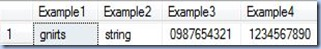## 05 December 2010

### A Look into Reverse

Some time back I was mentioning Stuff as one of the functions rarely used in SQL Server. Here’s another one: Reverse. I remember I used such a function in other programming languages and maybe 1-2 times in SQL Server, and I can’t say I seen it in action in other posts. As it name states and the MSDN documentation confirms, Reverse returns the reverse of a string value. The function takes as parameter any string value or value that could be implicitly conversed to a string, otherwise you’ll have to do an explicit conversion. As can be seen from the below first example the reverse of the “string” string is “gnirts”, and, as per the second example, the reverse of the revers of a string is the initial string itself. The third and fourth example use in exchange a numeric value.

```-- reversing a string using Reverse function
SELECT Reverse('string') Example1
, Reverse(Reverse('string')) Example2
, Reverse(1234567890) Example3
, Reverse(Reverse(1234567890)) Example4
```It seems there is not much to be said about Reverse function, and in the few situations that you need it, it’s great to have it, otherwise you would have to built it yourself. Let’s try to imagine there is no Reverse function in SQL Server, how would we provide such functionality by using existing functionality? In general such an exercise might look like lost time, though it could be useful in order to present several basic techniques. In this case the Reverse functionality could be provided by using a simple loop that iterates through string’s characters changing their order. The below piece of code should return the same output as above:

```-- reversing a string using looping
DECLARE @String varchar(50)
DECLARE @Retval varchar(50)
DECLARE @Index int
SET @String = 'string'
SET @Index = 1
SET @Retval = ''
WHILE @Index &lt;= Len(@String)
BEGIN
SET @Retval = Substring(@String, @Index, 1) + @Retval    SET @Index = @Index + 1
END
SELECT @Retval
```

Some type of problems whose solution involve the sequential processing of values within loops could be solved also using recursive techniques (recursion) that involve the sequential processing of values in which the output of a given intermediary step is based on the previous step. Recursion imply a slightly different view of the same problem, it could prove itself to be maybe less efficient in some cases and quite a useful technique in the others. SQL Server provides two types of recursion, one at function level involving functions, stored procedures are triggers, respectively at query level implemented in common table expressions. Recursive functions, functions that call themselves, have been for quite a while in SQL Server though few of the database books I read really talk about them. Probably I will detail the topic in another post, though for the moment I will show only the technique at work. In this case the recursive approach is quite simple:

```-- reversing a string using a recursive function
CREATE FUNCTION dbo.ReverseString(
@string varchar(50))
RETURNS varchar(50)
AS
BEGIN
RETURN (CASE WHEN Len(@string)>1 THEN dbo.ReverseString( Right(@string, len(@string)-1)) + Left(@string, 1) ELSE @string END)
END ```
` `
```-- testing
SELECT dbo.ReverseString('string') Example1
, dbo.ReverseString(dbo.ReverseString('string')) Example2
```

Common table expressions (CTE), since their introduction with SQL Server 2005, became quite an important technique in processing hierarchical structures like BOMs, which involve the traversal of the whole tree. A sequence is actually a tree having the width of 1 node, so it seems like a CTE’s job:

```-- reversing a string using common table expression
DECLARE @String varchar(50)
SET @String = 'string'
;WITH CTE(String, [Index])
AS (     SELECT Cast('' as varchar(50)) String
, 1 [Index]
UNION ALL
SELECT Cast(Substring(@String, [Index], 1) + String as varchar(50)) String
, [Index] + 1 [Index]
FROM CTE
WHERE [Index]<=Len(@String)
) ```
```SELECT @String Reversed
FROM CTE
WHERE [Index]-1=Len(@String)
```

Unlike the previous two solutions, the CTE approach involves more work, work that maybe isn’t required just for the sake of using the technique. When multiple alternative approaches exist, I prefer using the (simplest) solution that offers the highest degree of flexibility. In this case is the loop, though in others it could be a recursive function or a CTE.